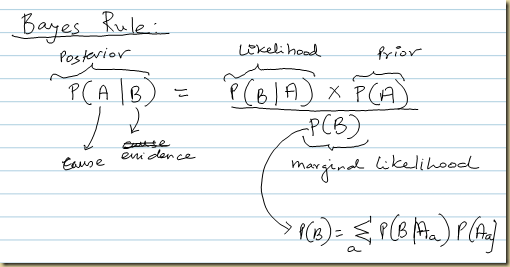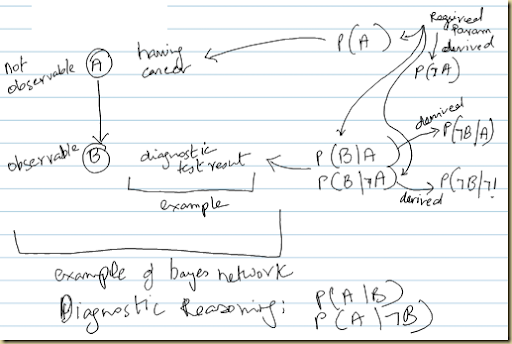## Saturday, October 22, 2011

### Bayes Network and Bayes Rule

As explained by Sebastin Thrun in the AI course:

Bayes Rule:Example:

Whats the likelihood that a person has cancer (A) given that they have had a positive cancer diagnostic test (B)?

• Likelihood: Probability of +ve cancer test (B) given that the person has cancer (A)
• Prior: Probability of getting that type of cancer (A)
• Marginal likelihood: Sum of probability of
(+ve test given that you have the cancer x probability of having that cancer)
(+ve test given that you don’t have the cancer (false positive) x probability of not having that cancer)

Bayes Network:Inferring P(B):

Wikipedia:

Baye’s Rule: http://en.wikipedia.org/wiki/Bayes_rule

Baye’s Network: http://en.wikipedia.org/wiki/Bayes_network